##Brianchon's theorem

In every circumscriptible in a circle hexagon, the diagonals, joining opposite vertices, pass through a common point O.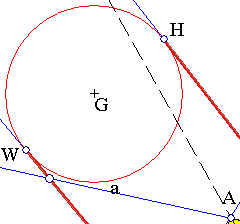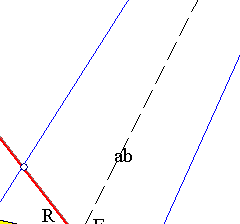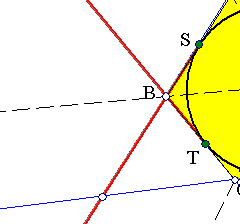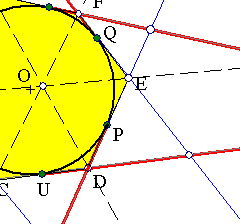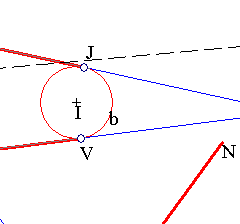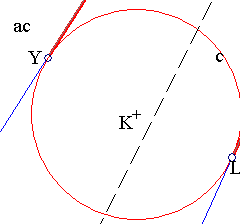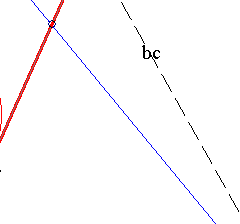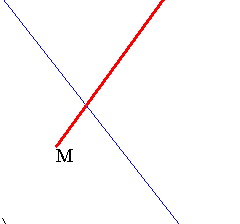To prove it take an arbitrary length (MN) and carry it on the tangents starting from the contact points: PL = RJ = QH = MN etc. Draw circles a, b, c tangent to opposite sides of the hexagon at the created points (H,W), (J,V) and (L,Y) respectively. One sees easily that the concurring lines coincide with the radical axes ab, bc, ca resepectively, of the three circles taken in pairs. Thus O coincides with the radical center of these three circles.

The theorem takes particular forms in the case of circumscriptible pentagons e.g. when R and Q tend to coincide with F, a case where AFE is transformed to the tangent at F. Then, taking a further similar indentification of points T,C and U, we obtain a corresponding theorem for quadrangles. These particular cases are examined in Brianchon2.html .

Notice that the theorem is the (projectively) dual of the theorem of Pascal and is valid for hexagons circumscriptible in conics. Look at Brianchon3.html for a picture.